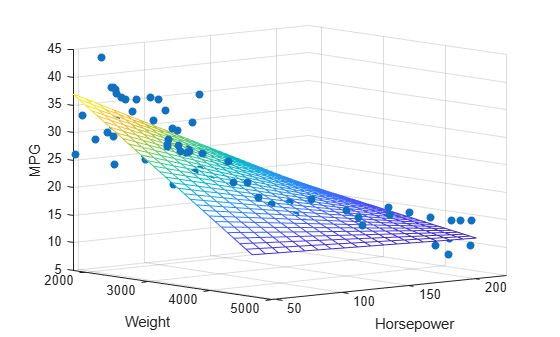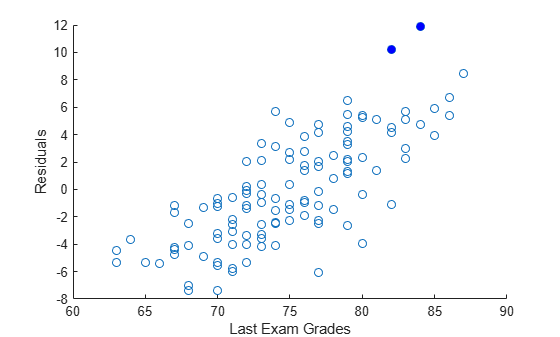Main Content

# regress

Multiple linear regression

## Syntax

``b = regress(y,X)``
``[b,bint] = regress(y,X)``
``[b,bint,r] = regress(y,X)``
``[b,bint,r,rint] = regress(y,X)``
``[b,bint,r,rint,stats] = regress(y,X)``
``[___] = regress(y,X,alpha)``

## Description

example

````b = regress(y,X)` returns a vector `b` of coefficient estimates for a multiple linear regression of the responses in vector `y` on the predictors in matrix `X`. To compute coefficient estimates for a model with a constant term (intercept), include a column of ones in the matrix `X`.```
````[b,bint] = regress(y,X)` also returns a matrix `bint` of 95% confidence intervals for the coefficient estimates.```
````[b,bint,r] = regress(y,X)` also returns an additional vector `r` of residuals.```

example

````[b,bint,r,rint] = regress(y,X)` also returns a matrix `rint` of intervals that can be used to diagnose outliers.```

example

````[b,bint,r,rint,stats] = regress(y,X)` also returns a vector `stats` that contains the R2 statistic, the F-statistic and its p-value, and an estimate of the error variance. The matrix `X` must include a column of ones for the software to compute the model statistics correctly.```

example

````[___] = regress(y,X,alpha)` uses a `100*(1-alpha)`% confidence level to compute `bint` and `rint`. Specify any of the output argument combinations in the previous syntaxes.```

## Examples

collapse all

Load the `carsmall` data set. Identify weight and horsepower as predictors and mileage as the response.

```load carsmall x1 = Weight; x2 = Horsepower; % Contains NaN data y = MPG;```

Compute the regression coefficients for a linear model with an interaction term.

```X = [ones(size(x1)) x1 x2 x1.*x2]; b = regress(y,X) % Removes NaN data```
```b = 4×1 60.7104 -0.0102 -0.1882 0.0000 ```

Plot the data and the model.

```scatter3(x1,x2,y,'filled') hold on x1fit = min(x1):100:max(x1); x2fit = min(x2):10:max(x2); [X1FIT,X2FIT] = meshgrid(x1fit,x2fit); YFIT = b(1) + b(2)*X1FIT + b(3)*X2FIT + b(4)*X1FIT.*X2FIT; mesh(X1FIT,X2FIT,YFIT) xlabel('Weight') ylabel('Horsepower') zlabel('MPG') view(50,10) hold off```Load the `examgrades` data set.

`load examgrades`

Use the last exam scores as response data and the first two exam scores as predictor data.

```y = grades(:,5); X = [ones(size(grades(:,1))) grades(:,1:2)];```

Perform multiple linear regression with alpha = 0.01.

`[~,~,r,rint] = regress(y,X,0.01);`

Diagnose outliers by finding the residual intervals `rint` that do not contain 0.

```contain0 = (rint(:,1)<0 & rint(:,2)>0); idx = find(contain0==false)```
```idx = 2×1 53 54 ```

Observations `53` and `54` are possible outliers.

Create a scatter plot of the residuals. Fill in the points corresponding to the outliers.

```hold on scatter(y,r) scatter(y(idx),r(idx),'b','filled') xlabel("Last Exam Grades") ylabel("Residuals") hold off```Load the `hald` data set. Use `heat` as the response variable and `ingredients` as the predictor data.

```load hald y = heat; X1 = ingredients; x1 = ones(size(X1,1),1); X = [x1 X1]; % Includes column of ones```

Perform multiple linear regression and generate model statistics.

`[~,~,~,~,stats] = regress(y,X)`
```stats = 1×4 0.9824 111.4792 0.0000 5.9830 ```

Because the ${R}^{2}$ value of `0.9824` is close to 1, and the p-value of `0.0000` is less than the default significance level of 0.05, a significant linear regression relationship exists between the response `y` and the predictor variables in `X`.

## Input Arguments

collapse all

Response data, specified as an n-by-1 numeric vector. Rows of `y` correspond to different observations. `y` must have the same number of rows as `X`.

Data Types: `single` | `double`

Predictor data, specified as an n-by-p numeric matrix. Rows of `X` correspond to observations, and columns correspond to predictor variables. `X` must have the same number of rows as `y`.

Data Types: `single` | `double`

Significance level, specified as a positive scalar. `alpha` must be between 0 and 1.

Data Types: `single` | `double`

## Output Arguments

collapse all

Coefficient estimates for multiple linear regression, returned as a numeric vector. `b` is a p-by-1 vector, where p is the number of predictors in `X`. If the columns of `X` are linearly dependent, `regress` sets the maximum number of elements of `b` to zero.

Data Types: `double`

Lower and upper confidence bounds for coefficient estimates, returned as a numeric matrix. `bint` is a p-by-2 matrix, where p is the number of predictors in `X`. The first column of `bint` contains lower confidence bounds for each of the coefficient estimates; the second column contains upper confidence bounds. If the columns of `X` are linearly dependent, `regress` returns zeros in elements of `bint` corresponding to the zero elements of `b`.

Data Types: `double`

Residuals, returned as a numeric vector. `r` is an n-by-1 vector, where n is the number of observations, or rows, in `X`.

Data Types: `single` | `double`

Intervals to diagnose outliers, returned as a numeric matrix. `rint` is an n-by-2 matrix, where n is the number of observations, or rows, in `X`. If the interval `rint(i,:)` for observation `i` does not contain zero, the corresponding residual is larger than expected in `100*(1-alpha)`% of new observations, suggesting an outlier. For more information, see Algorithms.

Data Types: `single` | `double`

Model statistics, returned as a numeric vector including the R2 statistic, the F-statistic and its p-value, and an estimate of the error variance.

• `X` must include a column of ones so that the model contains a constant term. The F-statistic and its p-value are computed under this assumption and are not correct for models without a constant.

• The F-statistic is the test statistic of the F-test on the regression model. The F-test looks for a significant linear regression relationship between the response variable and the predictor variables.

• The R2 statistic can be negative for models without a constant, indicating that the model is not appropriate for the data.

Data Types: `single` | `double`

## Tips

• `regress` treats `NaN` values in `X` or `y` as missing values. `regress` omits observations with missing values from the regression fit.

## Algorithms

collapse all

### Residual Intervals

In a linear model, observed values of `y` and their residuals are random variables. Residuals have normal distributions with zero mean but with different variances at different values of the predictors. To put residuals on a comparable scale, `regress` “Studentizes” the residuals. That is, `regress` divides the residuals by an estimate of their standard deviation that is independent of their value. Studentized residuals have t-distributions with known degrees of freedom. The intervals returned in `rint` are shifts of the `100*(1-alpha)`% confidence intervals of these t-distributions, centered at the residuals.

## Alternative Functionality

`regress` is useful when you simply need the output arguments of the function and when you want to repeat fitting a model multiple times in a loop. If you need to investigate a fitted regression model further, create a linear regression model object `LinearModel` by using `fitlm` or `stepwiselm`. A `LinearModel` object provides more features than `regress`.

• Use the properties of `LinearModel` to investigate a fitted linear regression model. The object properties include information about coefficient estimates, summary statistics, fitting method, and input data.

• Use the object functions of `LinearModel` to predict responses and to modify, evaluate, and visualize the linear regression model.

• Unlike `regress`, the `fitlm` function does not require a column of ones in the input data. A model created by `fitlm` always includes an intercept term unless you specify not to include it by using the `'Intercept'` name-value pair argument.

• You can find the information in the output of `regress` using the properties and object functions of `LinearModel`.

Output of `regress`Equivalent Values in `LinearModel`
`b`See the `Estimate` column of the `Coefficients` property.
`bint`Use the `coefCI` function.
`r`See the `Raw` column of the `Residuals` property.
`rint`Not supported. Instead, use studentized residuals (`Residuals` property) and observation diagnostics (`Diagnostics` property) to find outliers.
`stats`See the model display in the Command Window. You can find the statistics in the model properties (`MSE` and `Rsquared`) and by using the `anova` function.

 Chatterjee, S., and A. S. Hadi. “Influential Observations, High Leverage Points, and Outliers in Linear Regression.” Statistical Science. Vol. 1, 1986, pp. 379–416.

Download ebook